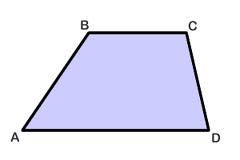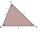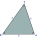# Trapezoid

Calculate area of trapezoid ABCD with sides |AD|= 68 cm, |DC|=35 cm, |CB|=12 cm, |AB|=35 cm..

Correct result:

A =  840 cm2

#### Solution:We would be pleased if you find an error in the word problem, spelling mistakes, or inaccuracies and send it to us. Thank you!Tips to related online calculators
Do you have a linear equation or system of equations and looking for its solution? Or do you have quadratic equation?

#### You need to know the following knowledge to solve this word math problem:

We encourage you to watch this tutorial video on this math problem:

## Next similar math problems:

• Is right triangle or notIf right triangle ABC, have sides a=13, b=11.5, c=22.5. Find area.
• Triangular prismCalculate the surface of a triangular prism 10 cm high, the base of which is a triangle with sides 6 cm 8 cm and 8 cm
• Find the areaFind the area of the triangle with the given measurements. Round the solution to the nearest hundredth if necessary. A = 50°, b = 30 ft, c = 14 ft
• Vertex pointsGiven the following points of a triangle: P(-12,6), Q(4,0), R(-8,-6). Graph the triangle. Find the triangle area.
• Compute 4Compute the exact value of the area of the triangle with sides 14 mi, 12 mi, and 12 mi long.
• The farmerThe farmer would like to first seed his small field. The required amount depends on the seed area. Field has a triangular shape. The farmer had fenced field, so he knows the lengths of the sides: 119, 111 and 90 meters. Find a suitable way to determine th
• Sides of the triangleCalculate triangle sides where its area is S = 84 cm2 and a = x, b = x + 1, xc = x + 2
• Circular railwayThe railway is to interconnect in a circular arc the points A, B, and C, whose distances are | AB | = 30 km, AC = 95 km, BC | = 70 km. How long will the track from A to C?
• One trapeziumOne trapezium has AB=24M, BC=36M, CD=80M, DA=80M long sides. Find the area.
• GardensThe area of the square garden is 3/4 of the area of the triangular garden with sides of 80 m, 50 m, 50 m. How many meters of the fence do we need to fence a square garden?What is the volume of a quadrilateral oblique prism with base edges of length a = 1m, b = 1.1m, c = 1.2m, d = 0.7m, if a side edge of length h = 3.9m has a deviation from the base of 20° 35 ´ and the edges a, b form an angle of 50.5°.Determine whether a triangle can be formed with the given side lengths. If so, use Heron's formula to find the area of the triangle. a = 158 b = 185 c = 201Calculate the size of all sides and internal angles of a triangle ABC, if it is given by area S = 501.9; and two internal angles α = 15°28' and β = 45°.Calculate the content of the area bounded by a circle circumscribed and a circle inscribed by a triangle with sides a = 25mm, b = 29mm, c = 36mmTrapezoid is given by length of four sides: 40.5 42.5 52.8 35.0. Calculate its area.Calculate the area and heights in the triangle ABC by sides a = 8cm, b = 11cm, c = 12cmCalculate the circumference of a triangle with area 84 cm2 if a:b:c = 10:17:21